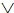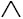# Boolean Algebra Questions and AnswersWhat is Boolean Algebra?

Boolean algebra, a method for applying and studying mathematical logic, was created by George Boolean, an English mathematician. He wrote “An investigation into the law of thought” in 1854. This book outlined a theory of logic that uses symbols rather than words. Boolean algebra is a more algebraic approach to the subject.

#### Questions on Boolean Algebra Mathematics with answers:

In Boolean Algebra, the zero element ‘0’
(a) Has two values
(b) Is unique
(c) As atleast two values
(d) None of these

Ans. (b)

Let B={p, q, r, …..} and let two binary operations be denoted by ‘‘ and ‘’ or ‘+’ or ‘.’, then
(a) 0’ = 0
(b) 0’ = 1
(c) 1’ = 1
(d) None of these

Ans. (b)

In Boolean Algebra, the unit element ‘1’
(a) Has two values
(b) Is unique
(c)

Has atleast two values
(d) None of these

Ans. (b)

Related: Biology Quiz: Endocrine System

In a Boolean Algebra B, for all x in B, xx =
(a) 0
(b) 1
(c) x
(d) None of these

Ans. (c)

In a Boolean Algebra B, for all x in B, xx =
(a) 0
(b) 1
(c) x
(d) None of these

Ans. (c)

Let B = {p, q, r, …..} and let two binary operations be denoted by ‘‘ and ‘’ or ‘+’ or ‘.’, then
(a) aa = 0
(b) aa = a
(c) a1 = a
(d) None of these

Ans. (b)

In a Boolean Algebra B, for all x in B,x1 =
(a) 0
(b) 1
(c) x
(d) None of these

Ans. (b)

In a Boolean Algebra B, for all x, y in B, x(xy) =
(a) y
(b) x
(c) 1
(d) 0

Ans. (b)

Let B={p, q, r, …..} and let two binary operations be denoted by ‘‘ and ‘’ or ‘+’ or ‘.’, then
(a) a(ab) = a
(b) a(ab) = b
(c) a(ab) = ab
(d) None of these

Ans. (a)

In a Boolean Algebra B, for all x, y in B, x(xy) =
(a) y
(b) x
(c) 1
(d) 0

Ans. (b)

In a Boolean Algebra B, for all x in B, (x’)’ =
(a) x
(b) x
(c) 1
(d) 0

Ans. (b)

In a Boolean Algebra B, for all x, y in B,(xy)’ =
(a) x’ v y
(b) xy
(c) 1
(d) None of these

Ans. (b)

In a Boolean Algebra B, for all x, y in B, (xy)’ =
(a) x’y’
(b) x’y’
(c) 1
(d) None of these

Ans. (b)

In a Boolean Algebra B, for all x in B, 1’ =
(a) 0
(b) 1
(c) x1
(d) None of these

Ans. (a)

Related: Newton’s Laws of Motion

Dual of (x’y’) = xy is
(a) (x’y’) = xy
(b) (x’y’) = xy
(c) (x’y’)’ = xy
(d) None of these

Ans. (b)

Dual of x(yx) = x is
(a) x(yx) = x
(b) x(yx) = x
(c) (xy) A(xx) = x
(d) None of these

Ans. (a)